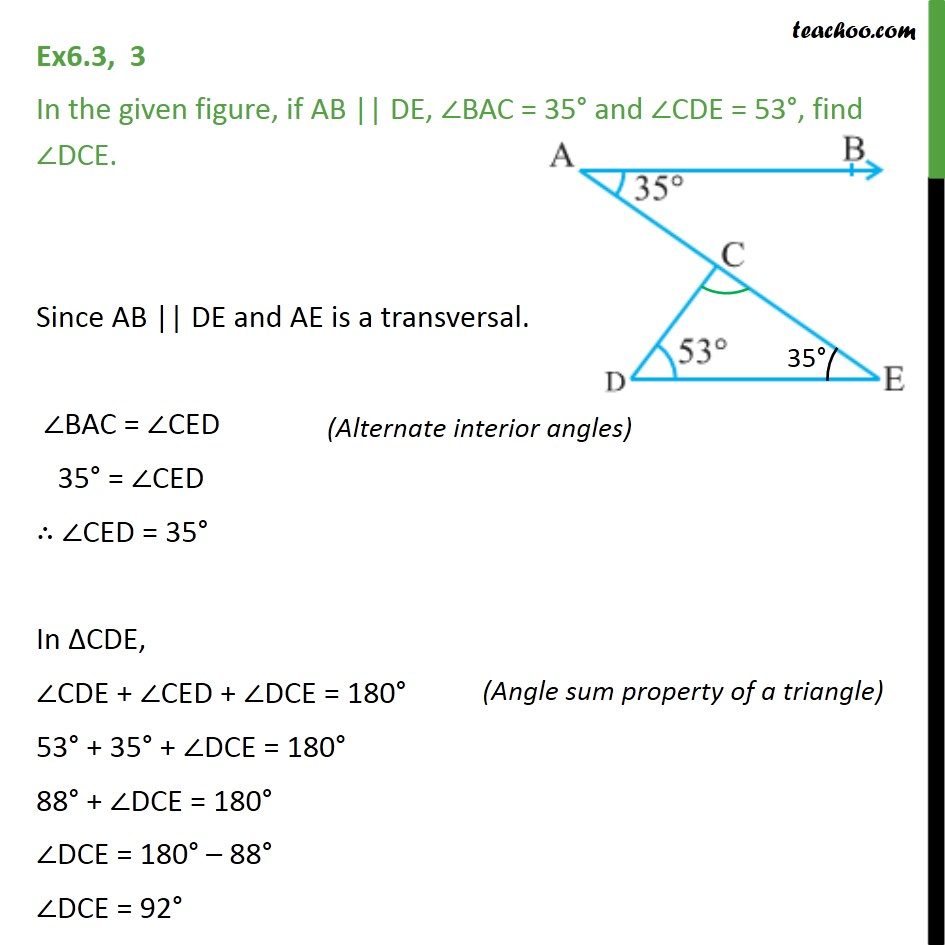1. Chapter 6 Class 9 Lines and Angles (Term 1)
2. Serial order wise
3. Ex 6.3

Transcript

Ex6.3, 3 In the given figure, if AB || DE, BAC = 35 and CDE = 53 , find DCE. Since AB || DE and AE is a transversal. BAC = CED 35 = CED CED = 35 In CDE, CDE + CED + DCE = 180 53 + 35 + DCE = 180 88 + DCE = 180 DCE = 180 88 DCE = 92